##### Common Core Math For Parents For Dummies with Videos Online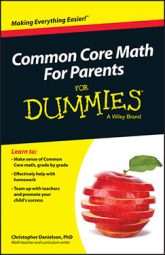Sixth graders solve a variety of Common Core math problems using ratios. The words ratio and rate are both appropriate in sixth grade and can mostly be used interchangeably.

A ratio is a comparison of two numbers of the same type (unit). Usually, ratio is used for part‐part comparisons, but not always. The word rate makes most people think about change, but a rate is a comparison of two numbers of different types (or units). Most people use the term ratio in situations where the numbers don't change and use rate when the numbers are changing.

Examples of rates that students work with include

• Dollars per hour

• Miles per hour

• Dollars per pound

• Students per class

Sixth graders develop a variety of strategies for solving ratio and rate problems. For an example, consider this problem:

The ratio of boys to girls in Ms. Wales's class is 3:2. If there are 30 students in the class, how many are boys and how many are girls?

Students may solve this problem with a ratio table, as shown in the figure.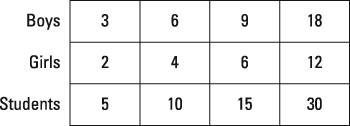A ratio table.

In a ratio table, a student keeps track of different equivalent forms of the ratio. The student may double (or triple, and so on) each value in the ratio to get larger values or may halve (or cut in three, and so on) each value to get smaller values. No two ratio tables for the same problem need to look identical, but they do need to maintain the given ratio throughout.

A student may notice that if there are 3 boys for every 2 girls, then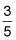of the students are boys. She may write the following series of fractions in order to figure out the right number of boys for a 30-student class. In this case, each fraction represents the part of the class that is boys: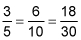The last fraction shows that 18 out of 30 students are boys in this scenario.

The important thing about ratios is that they are multiplication‐based comparisons of two numbers. If you double both numbers in a ratio — say 3:2 becomes 6:4 — the multiplication relationship between the numbers stays the same. In this example, 6 is still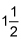times as big as 4, just as 3 isas big as 2.